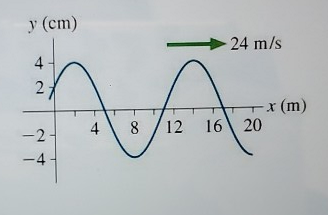# Problem: The figure below is a snapshot graph of a wave at t = 0s. What are the amplitude, wavelength, and frequency of the wave assuming that we know that this wave is travelling at 24 m/s in the positive x-direction?

###### FREE Expert Solution

In this problem, we are dealing with the properties of a wave.

93% (298 ratings)###### Problem Details

The figure below is a snapshot graph of a wave at t = 0s. What are the amplitude, wavelength, and frequency of the wave assuming that we know that this wave is travelling at 24 m/s in the positive x-direction?